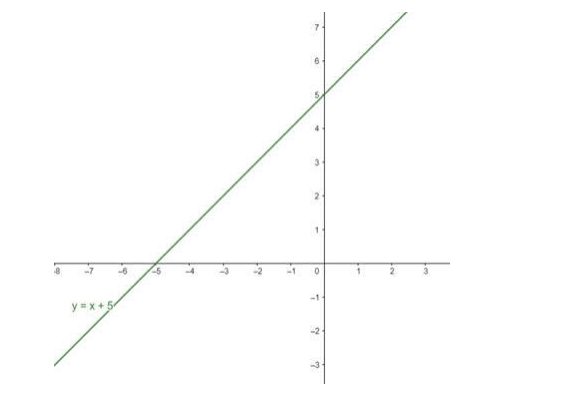# Consider the real function

Question:

Consider the real function $\mathrm{f}: \mathrm{R} \rightarrow \mathrm{R}: \mathrm{f}(\mathrm{x})=\mathrm{x}+5$ for all

$x \in R$. Find its domain and range. Draw the graph of this function.

Solution:

Given: $f(x)=x+5 \forall x \in R$

To Find: Domain and Range of f(x).

The domain of the given function is all real numbers expect where the expression is undefined. In this case, there is no real number which makes the expression undefined.

As f(x) is a polynomial function, we can have any value of x.

Therefore,

Domain $(f)=(-\infty, \infty)\{x \mid x \in R\}$

Now

Let $y=f(x)$

$y=x+5$

$x=y-5$

The range is set of all valid values of y

Therefore,

Range $(f)=(-\infty, \infty)\{y \mid y \in R\}$

Graph: